# mne.viz.plot_sparse_source_estimates#

mne.viz.plot_sparse_source_estimates(src, stcs, colors=None, linewidth=2, fontsize=18, bgcolor=(0.05, 0, 0.1), opacity=0.2, brain_color=(0.7, 0.7, 0.7), show=True, high_resolution=False, fig_name=None, fig_number=None, labels=None, modes=('cone', 'sphere'), scale_factors=(1, 0.6), verbose=None, **kwargs)[source]#

Plot source estimates obtained with sparse solver.

Active dipoles are represented in a “Glass” brain. If the same source is active in multiple source estimates it is displayed with a sphere otherwise with a cone in 3D.

Parameters:
src`dict`

The source space.

stcsinstance of `SourceEstimate` or `list` of instances of `SourceEstimate`

The source estimates.

colors`list`

List of colors.

linewidth`int`

Line width in 2D plot.

fontsize`int`

Font size.

bgcolor`tuple` of length 3

Background color in 3D.

opacity`float` in [0, 1]

Opacity of brain mesh.

brain_color`tuple` of length 3

Brain color.

show`bool`

Show figures if True.

high_resolution`bool`

If True, plot on the original (non-downsampled) cortical mesh.

fig_name`str`

PyVista figure name.

fig_number`int`

Matplotlib figure number.

labels

Labels to show sources in clusters. Sources with the same label and the waveforms within each cluster are presented in the same color. labels should be a list of ndarrays when stcs is a list ie. one label for each stc.

modes`list`

Should be a list, with each entry being `'cone'` or `'sphere'` to specify how the dipoles should be shown. The pivot for the glyphs in `'cone'` mode is always the tail whereas the pivot in `'sphere'` mode is the center.

scale_factors`list`

List of floating point scale factors for the markers.

verbose

Control verbosity of the logging output. If `None`, use the default verbosity level. See the logging documentation and `mne.verbose()` for details. Should only be passed as a keyword argument.

**kwargskwargs

Keyword arguments to pass to renderer.mesh.

Returns:
surfaceinstance of `Figure3D`

The 3D figure containing the triangular mesh surface.

## Examples using `mne.viz.plot_sparse_source_estimates`#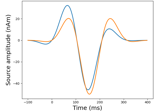Generate simulated evoked data

Generate simulated evoked data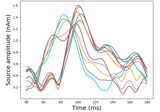Source localization with a custom inverse solver

Source localization with a custom inverse solver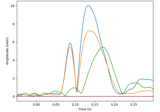Compute a sparse inverse solution using the Gamma-MAP empirical Bayesian method

Compute a sparse inverse solution using the Gamma-MAP empirical Bayesian method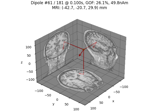Compute sparse inverse solution with mixed norm: MxNE and irMxNE

Compute sparse inverse solution with mixed norm: MxNE and irMxNE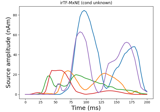Compute iterative reweighted TF-MxNE with multiscale time-frequency dictionary

Compute iterative reweighted TF-MxNE with multiscale time-frequency dictionary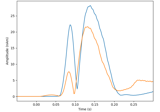Compute MxNE with time-frequency sparse prior

Compute MxNE with time-frequency sparse prior